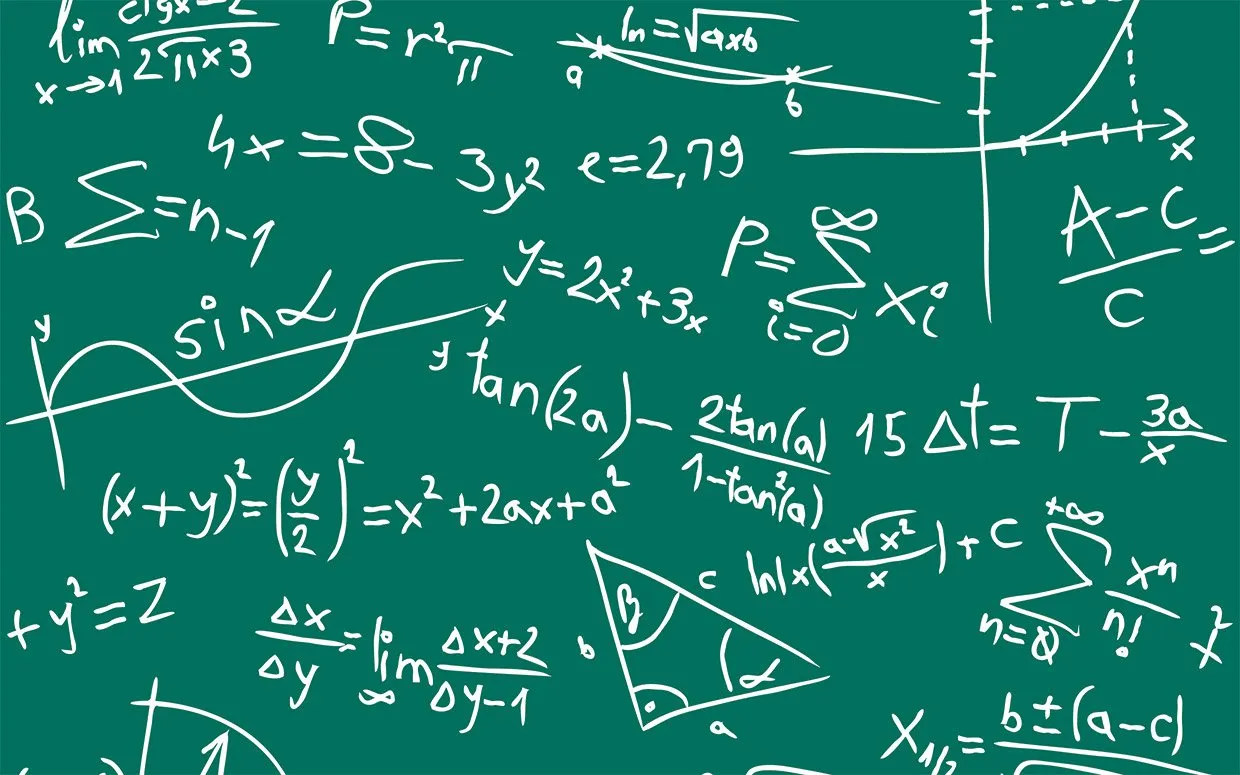# Mathematics - Ordinary first-order Differential equations (part 1)Heeeey it's me again drifter1. Today we continue with my Mathematics series to talk about Ordinary first-order DE's. Because there are 6 basic types of them we will cover 3 in this post and the other 3 the next time!

So, without further do, let's get started!

## First-order ODE types

Before getting into how we solve them we must talk about which types/categories of ODE's we have and can solve using simple methods.

A First-order ODE looks like this:

F(x, y, y') = 0 or y' = f(x, y)

And so contains only:

• the variable x
• function y with the first derivative (y') of it.

### Other notation

Another way of noting a Differential equations is using the differentials dx, dy.

Using this notation we can distinguish some types better and it's not so difficult to go from one notation to another anyway (I will show you what I mean when we get into examples).

### Types

Depending on the way we order the x, y and y' parts, mostly separating them because of the coefficients, we get 6 basic types of first-order ODE's.

Those are:

1. Separable -> P(x)*dx + Q(y)*dy = 0
2. Homogeneous -> y' = P(x,y) / Q(x,y), where the quotient P/Q is homogeneous of 0-order
3. Linear -> y' + P(x)*y = Q(x)
4. Bernoulli -> y' + P(x)*y + Q(x)*y^a = 0, where a != 0, 1 and real.
5. Riccati -> y' + P(x)*y^2 + Q(x)*y + R(x) = 0
6. Exact -> P(x, y)*dx + Q(x, y)*dy = 0, where P'y == Q'x (partial derivatives)

Let's now get into how we solve 1, 2 and 6.

## Solving Separable ODE's

A separable variable ODE is of the form:

P(x) dx + Q(y)dy = 0

We can see that we can separate the variable x, y to be in one side of the equation.

By doing this we get:

integral[P(x)dx] = integral[-Q(y)dy]

From this equation we will get our final solution.

## Solving Homogeneous ODE's

To solve a homogeneous of the form:

y' = P(x,y) / Q(x,y)

we must convert it into a separable-type one.

To do this we must first check if it's really homogeneous.

P(x, y) / Q(x,y) must equal P(λx, λy) / Q(λx, λy)

And so P(λx, λy) = λ^n*P(x,y) and Q(λx, λy) = λ^n*Q(x, y)

If that's true then the quotient is 0-order homegeneous and the ODE is homogeneous.

### Solving

To solve it we must find a function u(x, y) by doing calculations on the actual ODE to find some connection between x, y.

For example we could have a lot of y/x parts and only x's alone.

By setting u(x, y) = y/x we get a ODE that contains only u and x.

y = ux => y'(ux)' = u'x + u => ... will give us a separable

## Solving Exact ODE's

There is a small problem here. I  have not covered partial derivatives in Mathematical Analysis, but I hope that this will not be such a big problem.

When partial derivating a function that contains to variables x, y we derivative using one of the two variables (x or y) and think of the other like a constant c.

The form of an exact is:

P(x, y)*dx + Q(x, y)*dy = 0

That way P(x, y) will be partially differentiated using y and Q(x, y) the same using x.

If the result is the same (Py = Qx)  then the ODE is exact, else it's not and we must convert it into an exact by using an integrating factor.

### Converting into exact

To convert it we multiply our given ODE with a function μ(x, y).

There are 3 basic forms of μ that depend on some quotient:

1. If (Py - Qx) / Q: function of x then μ = e^integral[(Py-Qx)/Q]dx
2. If (Qx - Py) / P: function of y then μ = e^integral[(Qx - Py) / P]dy
3. If (Py - Qx)/(yQ - xP): function of w=xy then μ = e^integral[(Py - Qx)/(yQ - xP)]d(xy)

### Solving

If exact we do the following steps:

f(x, y) = integral[P(x, y)dx] = f1(x, y) + c1(y)     (partial integrate using x)

f'y = Q(x, y) => f1'y(x, y) + c1'y = Q(x, y) => c1(y) = integral[Q(x, y) - f1'(x, y)] dy

The partial derivative of f with is equal to Q(x, y).

Using the first equation for the left part and solving for c1(y) we get an integral that will give us c1(y).

By setting c1(y) to this result in the first equation we get our final solution.

### Image sources:### Previous post of the series:

Introduction -> Definition and Applications

And this is it for today!

I hope you enjoyed it and learned something new (or remembered).

Next time we will get into 3 more 1st-order ODE's.

Ciao!

H2
H3
H4
3 columns
2 columns
1 column